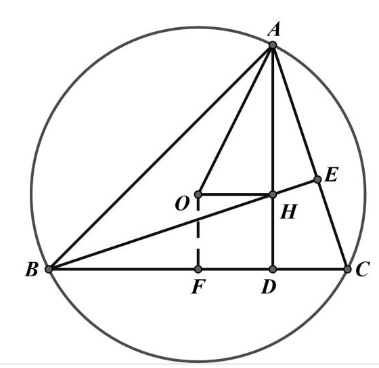【答案】

$\frac{2+x}{3}=\frac{1}{x}$, 解得 $x=1$
$A D=3$, 由 $O B=O A$ 得
$\sqrt{2^2+d^2}=\sqrt{(3-d)^2+1^2}$ 得 $d=1$
$\therefore O H D F$ 为正方形, $O H=1$